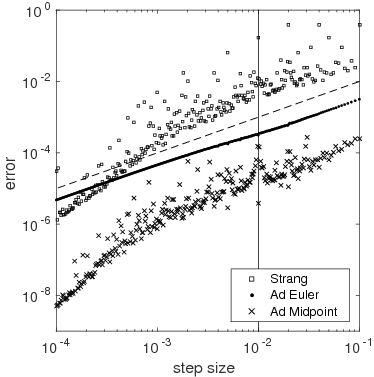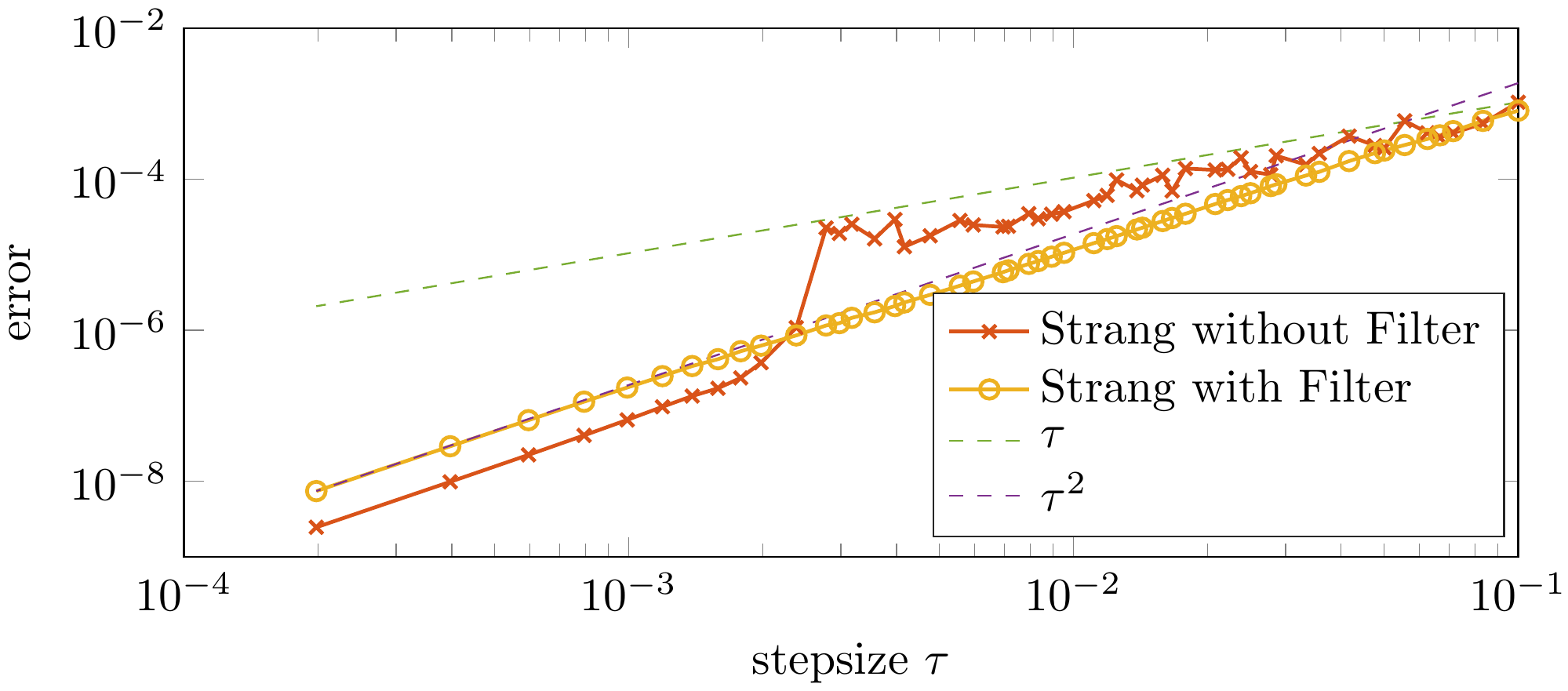# Project A7 • Numerical methods for highly oscillatory problems

### Principal investigators

 Prof. Dr. Marlis Hochbruck (7/2015 - ) Prof. Dr. Tobias Jahnke (7/2015 - ) Prof. Dr. Christian Lubich (7/2015 - )

### Project summary

Evolution equations with highly oscillatory solutions are abundant in the simulation of wave phenomena. When standard numerical integrators are applied to such problems, an acceptable accuracy can only be achieved if the size of the time steps is small compared to the inverse of the highest frequency arising in the system. As a consequence, many time steps have to be carried out, which incurs huge computational costs and thus makes traditional methods prohibitively inefficient. The central goals of this project are the construction, analysis, and efficient implementation of numerical integrators for highly oscillatory problems arising in simulations of wave phenomena. Our main objective is to prove error bounds which guarantee convergence of the method under realistic regularity assumptions and without any severe step size restrictions.

• Adiabatic integrators for dispersion-managed nonlinear Schrödinger equations.

Data transmission through an optical fiber with strong dispersion management is modeled by the semilinear Schrödinger equation \begin{equation}\label{DMNLS} \partial_t u(t,x) = \tfrac{\mathrm{i}}{\varepsilon} \gamma\left(\tfrac{t}{\varepsilon}\right) \partial_x^2 u(t,x)+\mathrm{i} |u(t,x)|^2 u(t,x)\,, \quad t\in[0,T]\,\end{equation} with a small parameter $0\lt\varepsilon\ll T$;[TBF12]. The coefficient function $\gamma$ is given by $\begin{equation}\label{gamma} \gamma(t) = \chi(t)+\varepsilon\alpha\,,\end{equation}$ where $\alpha\gt0$ is the mean dispersion, and where $\begin{equation}\label{chi} \chi(t) = \left\{\begin{array}{ll}-\delta\,, \text{ if } t \in [m, m+1) \text{ for even } m \in \mathbb{N}, \\[2mm] \,\,\,\,\delta\,, \text{ if } t \in [m, m+1) \text{ for odd }\, m \in \mathbb{N} \end{array}\right.\end{equation}$ is a periodic, piecewise constant function which alternates between $-\delta$ and $+\delta$. A short introduction to dispersion management from a mathematical perspective can be found in the introduction of [JM18] and in the dissertation [Mik17].

Approximating the solution of \eqref{DMNLS} numerically is a challenging task. The factor $\mathrm{i}/\varepsilon$ in the right-hand side causes a highly oscillatory behavior of typical solutions. As a consequencs, traditional numerical time-integrators (e.g. Runge-Kutta or multistep methods) yield a very poor accuracy unless a huge number of time-steps with a tiny step-size $\tau\ll\varepsilon$ is made, which causes excessive numerical costs. Additional difficulties are caused by the fact that the time-derivative $t \mapsto \partial_t u(t,\cdot)$ discontinuous due to the piecewise constant coefficient function $\gamma$. This is in contradiction to standard assumptions which are typically made to prove higher order convergence of many time integrators. Last but not least, the nonlinearity $\mathrm{i} |u(t,x)|^2 u(t,x)$ makes implicit methods prohibitively costly and complicates the construction of new integrators.

In [JM18] we have devised and analyzed a novel time-integrator for the dispersion-managed nonlinear Schrödinger equation \eqref{DMNLS} on the torus $\mathbb{T}=\mathbb{R}/2\pi\mathbb{Z}$. This new method, called the adiabatic midpoint rule, is based on a transformation of \eqref{DMNLS} to an evolution equation which has several advantageous properties, and on the fact that certain highly oscillatory exponential functions which occur in the transformed equation can be integrated analytically. This approach is related to the ideas developed in [Jah04, JL03]. It was shown that under suitable regularity assumptions the adiabatic midpoint rule converges with order 1 with an error constant which does not depend on $\varepsilon$ and without any $\varepsilon$-induced step-size restriction. Moreover, we proved that the accuracy increases if the step-size $\tau$ is chosen in a special way: The global error reduces to $\mathcal{O}(\varepsilon^2 + \tau^2)$ if $\tau = \varepsilon k$ with $k\in \mathbb{N}$, and to $\mathcal{O}(\varepsilon\tau)$ if $\tau = \varepsilon/k$, respectively. In both cases the error constant remains independent on $\varepsilon$, which explains the excellent accuracy of the adiabatic midpoint rule in numerical experiments; cf. Figure 1.Figure 1: Maximal $l^2$-error over time of the adiabatic midpoint rule for $\varepsilon=0.01$ (top), $\varepsilon=0.002$ (bottom). In the left panels the accuracy of the adiabatic Euler method and the Strang splitting is shown as reference. In the right panels only the accuracy for step-sizes of the form $\tau=\varepsilon k$ and $\tau=\varepsilon/k$ for $k\in\mathbb{N}$ is shown.

Unfortunately, the $L_2$ norm of numerical solutions provided by the adiabatic midpoint rule is in general not constant, although it can easily be shown that the norm $\|u(t,\cdot)\|_{L_2}$ of every solution of \eqref{DMNLS} is preserved. This was our motivation to construct an exponential version of the adiabatic midpoint rule which conserves the norm of the numerical solution exactly and, in addition, has the same favourable convergence behaviour as its non-exponential counterpart. The exponential adiabatic midpoint rule presented in [JM19] is closely related to our approach in [JM18], but in the error analysis we had to cope with new challenges arising from the exponential structure of the new method.

In mathematical physics the dispersion-managed nonlinear Schrödinger equation \eqref{DMNLS} is often considered on $\mathbb{R}$ instead of the torus $\mathbb{T}$. Unfortunately, extending the techniques developed in [JM19, Mik17, TBF12] from $\mathbb{R}$ to $\mathbb{T}$ is impossible, such that the construction of efficient numerical methods for \eqref{DMNLS} on $\mathbb{R}$ requires completely new ideas. Julian Baumstark (PhD student since 10/2017) and Tobias Jahnke are currently working on this problem.

• Splitting methods for highly oscillatory differential equations

Splitting methods have become an essential tool in solving (partial) differential equations numerically. For oscillatory differential equations, though, classical schemes are inefficient and suffer from order reduction since their solution does not fulfill the required high regularity assumptions. Hence we need to come up with different approaches that take the oscillatory nature of the problem into account.

Simulating phenomena like vibration and light is an omnipresent task in modern technology and research. From physics we have for example the discrete wave equation \begin{align} \label{eq: wave_discr} u''(t) = - \Omega^2 u(t) + g(u). \end{align} with a matrix $\Omega$ of extremely large norm while the nonlinearity $g$ is suitably smooth. Such solutions can be approximated by many well established numerical algorithms. For the analysis and construction the behaviour of the wave, so the solution $u$, needs to be somewhat “nice”, which means for example that higher derivatives of the solution have to exist and are suitably bounded.
From an analytic point of view we often cannot guarantee such regularity. Also numerical experiments show that for non smooth solutions resonances in the error can be observed if the step size times a high frequency of the system happens to hit a multiple of $2 \pi$.We now raise the question if there is a way to overcome these problems and still get reasonable approximations of the real wave?
In [GH06], [HLW06] several trigonometric integrators are proposed to solve such problems. They also note that those can be understood as a Splitting method for a filtered equation, where the nonlinearity $g$ is modified by filters depending on the oscillating part $\Omega$ and the step size $\tau$.
To gain a better insight, in [BGGHJ18] the analysis was done from this point of view. We considered the discrete wave equation (\ref{eq: wave_discr}) with a linear $g$ and integrated it using the Strang Splitting applied to the modified equation. Thereby it was proven that the error still is of order $\mathcal{O}(\tau^2)$. In a first step one estimates the error obtained by the perturbation through the filter. In a second step the error of the splitting is analysed. Because of the filters some critical terms can be handled and one can partly use the classical techniques for Splitting methods. Arguments like the lady windermere's fan, though, need to be carefully adapted to this special situation using for example summation by parts. The results can also be extended to nonlinear functions $g$.
We now want to generalize the results. We take a step back from the ODE level to the abstract evolution equation arising from \begin{align*} u''(t) = \Delta u(t) + f(u) \end{align*}. On the one hand this will widen the range of possible equations we can treat, on the other hand we will gain deeper insights in the behaviour of the numerical scheme which will hopefully lead to the right ideas for analysing the full discretisation error.

## Publications

1. . A conjugate-gradient-type rational Krylov subspace method for ill-posed problems. Inverse Problems, 36(1):015008, . [preprint] [bibtex]

2. and . Adiabatic exponential midpoint rule for the dispersion-managed nonlinear Schrödinger equation. IMA J. Numer. Anal., 39(4):1818–1859, October . [preprint] [bibtex]

3. , , and . Heterogeneous multiscale method for Maxwell's equations. Multiscale Model. Simul., 17(4):1147–1171, October . [preprint] [bibtex]

4. and . Adiabatic midpoint rule for the dispersion-managed nonlinear Schrödinger equation. Numer. Math., 138(4):975–1009, April . [preprint] [bibtex]

5. , , , , and . Closing the gap between trigonometric integrators and splitting methods for highly oscillatory differential equations. IMA J. Numer. Anal., 38(1):57–74, January . [preprint] [bibtex]

6. and . Finite element heterogeneous multiscale method for time-dependent Maxwell's equations. In M. L. Bittencourt, N. A. Dumont, and J. S. Hesthaven, editors, Spectral and high order methods for partial differential equations—ICOSAHOM 2016, volume 119 of Lect. Notes Comput. Sci. Eng., pages 269–281. Springer, Cham, November . [preprint] [bibtex]

7. , , and . On the approximation of electromagnetic fields by edge finite elements. Part 2: A heterogeneous multiscale method for Maxwell's equations. Comput. Math. Appl., 73(9):1900–1919, May . [preprint] [bibtex]

8. , , and . Long-term analysis of semilinear wave equations with slowly varying wave speed. Comm. Partial Differential Equations, 41(12):1934–1959, September . [preprint] [bibtex]

## Preprints

1. , , and . On averaged exponential integrators for semilinear wave equations with solutions of low-regularity. CRC 1173 Preprint 2020/8, Karlsruhe Institute of Technology, March . [bibtex]

2. , , and . On the convergence of Lawson methods for semilinear stiff problems. CRC 1173 Preprint 2019/20, Karlsruhe Institute of Technology, October . [bibtex]

3. and . Long-term analysis of a variational integrator for charged-particle dynamics in a strong magnetic field. CRC 1173 Preprint 2018/6, Karlsruhe Institute of Technology, April . [bibtex]

## Theses

1. . Fehleranalyse von auf trigonometrischen Integratoren basierenden Splittingverfahren für hochoszillatorische, semilineare Probleme. PhD thesis, Karlsruhe Institute of Technology (KIT), November . [bibtex]

2. . Time-integration methods for a dispersion-managed nonlinear Schrödinger equation. PhD thesis, Karlsruhe Institute of Technology (KIT), June . [bibtex]

## Other references

1. , , and . Dispersion-managed solitons in fibre systems and lasers. Phys. Rep., 521(4):135–203, December . [bibtex]

2. and . Error analysis of exponential integrators for oscillatory second-order differential equations. J. Phys. A, 39(19):5495–5507, April . [bibtex]

3. , , and . Geometric numerical integration, volume 31 of Springer Series in Computational Mathematics. Springer-Verlag, Berlin, Second edition, . Structure-preserving algorithms for ordinary differential equations. [bibtex]

4. . Long-time-step integrators for almost-adiabatic quantum dynamics. SIAM J. Sci. Comput., 25(6):2145–2164, July . [bibtex]

5. and . Numerical integrators for quantum dynamics close to the adiabatic limit. Numer. Math., 94(2):289–314, April . [bibtex]

Project-specific staff
NamePhoneE-Mail+49 721 608-43843 julian baumstarkRav7∂kit edu
+49 721 608-42683 constantin carleDhp8∂kit edu
+49 721 608-42771 volker grimmVgj5∂kit edu
+49 721 608-42060 marlis hochbruckVhd6∂kit edu
+49 721 608-47982 tobias jahnkeGzg4∂kit edu
+49 7071 29-72935 lubichKwx1∂na uni-tuebingen de
+49 721 608-42683 bernhard maierRfh1∂kit edu
+49 721 608-44342 stefan schrammerYvi5∂kit edu

Former staff
NameTitleFunctionDr. Doctoral researcher
Dr. Doctoral researcher and Postdoctoral researcher
Dr. Postdoctoral researcher